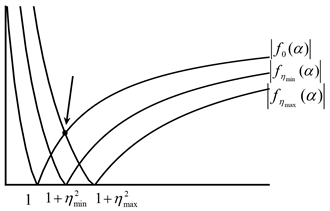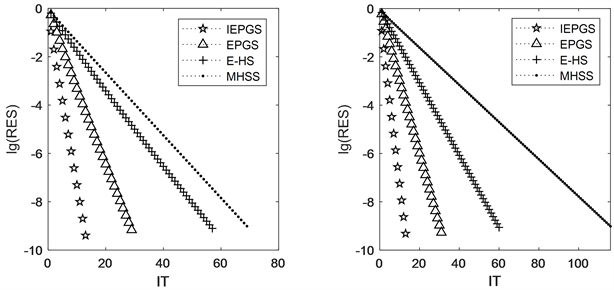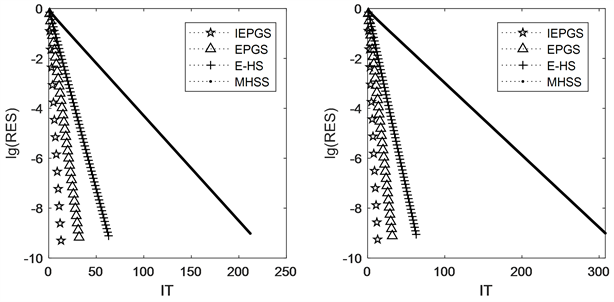﻿ 求解复对称线性系统的改进EPGS迭代法

# 求解复对称线性系统的改进EPGS迭代法Improved EPGS Iterative Method for Solving Complex Symmetric Linear Systems

Abstract: In this paper, we mainly discuss the EPGS iterative method for solving complex symmetric linear systems. Based on the idea of triangular splitting of matrix blocks, we introduce an acceleration parameter to this method and obtain the improved EPGS iterative method (IEPGS). We analyze the convergence of IEPGS iteration method, give the condition of convergence, and obtain the optimal iteration parameters and the corresponding optimal convergence factors. Numerical experiments show that the method is effective.

1. 引言

$Au=\left(W+\text{i}T\right)u=b$, $A\in {C}^{n×n}$, $W,T\in {R}^{n×n}$, $u,b\in {C}^{n}$ (1)

$Bz\equiv \left[\begin{array}{cc}W& -T\\ T& W\end{array}\right]\left[\begin{array}{c}x\\ y\end{array}\right]=\left[\begin{array}{c}f\\ g\end{array}\right]\equiv p$, $B\in {R}^{2n×2n}$, $z,p\in {R}^{2n}$, $x,y,f,g\in {R}^{n}$ (2)

2. IEPGS迭代法

${G}_{\theta }=\left[\begin{array}{cc}\mathrm{cos}\left(\theta \right)I& -\mathrm{sin}\left(\theta \right)I\\ \mathrm{sin}\left(\theta \right)I& \mathrm{cos}\left(\theta \right)I\end{array}\right]$,

$\stackrel{˜}{B}z={G}_{\theta }^{\text{T}}Bz=\left[\begin{array}{cc}{\stackrel{˜}{W}}_{\theta }& -{\stackrel{˜}{T}}_{\theta }\\ {\stackrel{˜}{T}}_{\theta }& {\stackrel{˜}{W}}_{\theta }\end{array}\right]\left[\begin{array}{c}x\\ y\end{array}\right]={G}_{\theta }^{\text{T}}\left[\begin{array}{c}f\\ g\end{array}\right]=\left[\begin{array}{c}\stackrel{˜}{f}\\ \stackrel{˜}{g}\end{array}\right]=\stackrel{˜}{p}$ (3)

$\left\{\begin{array}{l}{\stackrel{˜}{W}}_{\theta }{x}^{k+1}={\stackrel{˜}{T}}_{\theta }{y}^{k}+\stackrel{˜}{f},\\ {\stackrel{˜}{W}}_{\theta }{y}^{k+1}=-{\stackrel{˜}{T}}_{\theta }{x}^{k+1}+\stackrel{˜}{g}\end{array}$ (4)

$\stackrel{˜}{B}=\left[\begin{array}{cc}\alpha {\stackrel{˜}{W}}_{\theta }& 0\\ {\stackrel{˜}{T}}_{\theta }& {\stackrel{˜}{W}}_{\theta }\end{array}\right]-\left[\begin{array}{cc}\left(\alpha -1\right){\stackrel{˜}{W}}_{\theta }& {\stackrel{˜}{T}}_{\theta }\\ 0& 0\end{array}\right]=M\left(\theta ,\alpha \right)-N\left(\theta ,\alpha \right)$

$\left[\begin{array}{c}{x}^{\left(k+1\right)}\\ {y}^{\left(k+1\right)}\end{array}\right]=\Gamma \left(\theta ,\alpha \right)\left[\begin{array}{c}{x}^{\left(k\right)}\\ {y}^{\left(k\right)}\end{array}\right]+\varphi \left(\theta ,\alpha \right)\left[\begin{array}{c}\stackrel{˜}{f}\\ \stackrel{˜}{g}\end{array}\right]$, (5)

$\Gamma \left(\theta ,\alpha \right)=\left[\begin{array}{cc}\frac{1}{\alpha }{\stackrel{˜}{W}}_{\theta }^{-1}& 0\\ -\frac{1}{\alpha }{\stackrel{˜}{W}}_{\theta }^{-1}{\stackrel{˜}{T}}_{\theta }{\stackrel{˜}{W}}_{\theta }^{-1}& {\stackrel{˜}{W}}_{\theta }^{-1}\end{array}\right]\left[\begin{array}{cc}\left(\alpha -1\right){\stackrel{˜}{W}}_{\theta }& {\stackrel{˜}{T}}_{\theta }\\ 0& 0\end{array}\right]=\left[\begin{array}{cc}\left(1-\frac{1}{\alpha }\right)I& \frac{1}{\alpha }{\stackrel{˜}{W}}_{\theta }^{-1}{\stackrel{˜}{T}}_{\theta }\\ -\left(1-\frac{1}{\alpha }\right){\stackrel{˜}{W}}_{\theta }^{-1}{\stackrel{˜}{T}}_{\theta }& -\frac{1}{\alpha }{\stackrel{˜}{W}}_{\theta }^{-1}{\stackrel{˜}{T}}_{\theta }{\stackrel{˜}{W}}_{\theta }^{-1}{\stackrel{˜}{T}}_{\theta }\end{array}\right]$ (6)

$\left\{\begin{array}{l}\alpha {\stackrel{˜}{W}}_{\theta }{x}^{k+1}=\left(\alpha -1\right){\stackrel{˜}{W}}_{\theta }{x}^{k}+{\stackrel{˜}{T}}_{\theta }{y}^{k}+\stackrel{˜}{f},\\ {\stackrel{˜}{W}}_{\theta }{y}^{k+1}=-{\stackrel{˜}{T}}_{\theta }{x}^{k+1}+\stackrel{˜}{g}.\end{array}$ (7)

3. IEPGS迭代法的收敛性分析

1) 迭代矩阵 $\Gamma \left(\theta ,\alpha \right)$ 的特征值 $\lambda$ 取值如下：

$\lambda =0$, $\lambda =1-\frac{1}{\alpha }$, $\lambda =1-\frac{1+{\eta }_{i}^{2}}{\alpha }$ (8)

2) 谱半径 $\rho \left(\Gamma \left(\theta ,\alpha \right)\right)<1$ 当且仅当 $\alpha >\frac{1+{\eta }_{\mathrm{max}}^{2}}{2}$

3) $\rho \left(\Gamma \left(\theta ,\alpha \right)\right)$ 可表示为：

$\rho \left(\Gamma \left(\theta ,\alpha \right)\right)=\mathrm{max}\left\{|1-\frac{1+{\eta }_{\mathrm{max}}^{2}}{\alpha }|,|1-\frac{1+{\eta }_{\mathrm{min}}^{2}}{\alpha }|,|1-\frac{1}{\alpha }|\right\}$ (9)

${P}_{1}=\left[\begin{array}{cc}{\stackrel{˜}{W}}_{\theta }^{-\frac{1}{2}}& 0\\ 0& {\stackrel{˜}{W}}_{\theta }^{-\frac{1}{2}}\end{array}\right]$ (10)

$\stackrel{^}{\Gamma }\left(\theta ,\alpha \right)=\left[\begin{array}{cc}{\stackrel{˜}{W}}_{\theta }^{\frac{1}{2}}& 0\\ 0& {\stackrel{˜}{W}}_{\theta }^{\frac{1}{2}}\end{array}\right]\left[\begin{array}{cc}\left(1-\frac{1}{\alpha }\right)I& \frac{1}{\alpha }{\stackrel{˜}{W}}_{\theta }^{-1}{\stackrel{˜}{T}}_{\theta }\\ -\left(1-\frac{1}{\alpha }\right){\stackrel{˜}{W}}_{\theta }^{-1}{\stackrel{˜}{T}}_{\theta }& -\frac{1}{\alpha }{\stackrel{˜}{W}}_{\theta }^{-1}{\stackrel{˜}{T}}_{\theta }{\stackrel{˜}{W}}_{\theta }^{-1}{\stackrel{˜}{T}}_{\theta }\end{array}\right]\left[\begin{array}{cc}{\stackrel{˜}{W}}_{\theta }^{-\frac{1}{2}}& 0\\ 0& {\stackrel{˜}{W}}_{\theta }^{-\frac{1}{2}}\end{array}\right]=\left[\begin{array}{cc}\left(1-\frac{1}{\alpha }\right)I& \frac{1}{\alpha }Q\\ -\left(1-\frac{1}{\alpha }\right)Q& -\frac{1}{\alpha }{Q}^{2}\end{array}\right]$

$Q={U}^{\text{T}}\left[\begin{array}{cc}{\Sigma }_{r}& 0\\ 0& 0\end{array}\right]U$, (11)

${P}_{2}=\left[\begin{array}{cc}{U}^{\text{T}}& 0\\ 0& {U}^{\text{T}}\end{array}\right]$ (12)

$\stackrel{˜}{\Gamma }\left(\theta ,\alpha \right)=\left[\begin{array}{cc}U& 0\\ 0& U\end{array}\right]\left[\begin{array}{cc}\left(1-\frac{1}{\alpha }\right)I& \frac{1}{\alpha }Q\\ -\left(1-\frac{1}{\alpha }\right)Q& -\frac{1}{\alpha }{Q}^{2}\end{array}\right]\left[\begin{array}{cc}{U}^{\text{T}}& 0\\ 0& {U}^{\text{T}}\end{array}\right]=\left[\begin{array}{cc}\left(1-\frac{1}{\alpha }\right)I& \frac{1}{\alpha }UQ{U}^{\text{T}}\\ -\left(1-\frac{1}{\alpha }\right)UQ{U}^{\text{T}}& -\frac{1}{\alpha }UQ{U}^{\text{T}}UQ{U}^{\text{T}}\end{array}\right]$

$\stackrel{˜}{\Gamma }\left(\theta ,\alpha \right)=\left[\begin{array}{cccc}\left(1-\frac{1}{\alpha }\right){I}_{r}& 0& \frac{1}{\alpha }{\Sigma }_{r}& 0\\ 0& \left(1-\frac{1}{\alpha }\right){I}_{n-r}& 0& 0\\ -\left(1-\frac{1}{\alpha }\right){\Sigma }_{r}& 0& -\frac{1}{\alpha }{\Sigma }_{r}^{2}& 0\\ 0& 0& 0& 0\end{array}\right]$

$\begin{array}{c}|\lambda I-\stackrel{˜}{\Gamma }\left(\theta ,\alpha \right)|=|\begin{array}{cccc}\left(\lambda -1+\frac{1}{\alpha }\right){I}_{r}& 0& -\frac{1}{\alpha }{\Sigma }_{r}& 0\\ 0& \left(\lambda -1+\frac{1}{\alpha }\right){I}_{n-r}& 0& 0\\ \left(1-\frac{1}{\alpha }\right){\Sigma }_{r}& 0& \lambda {I}_{r}-\frac{1}{\alpha }{\Sigma }_{r}^{2}& 0\\ 0& 0& 0& \lambda {I}_{n-r}\end{array}|\\ ={\left(-1\right)}^{r}\cdot {\lambda }^{r}\cdot \left[\left(\lambda -1+\frac{1}{\alpha }\right)\frac{\alpha }{{\eta }_{1}}+{\eta }_{1}\right]\cdot \cdots \cdot \left[\left(\lambda -1+\frac{1}{\alpha }\right)\frac{\alpha }{{\eta }_{r}}+{\eta }_{r}\right]\cdot {\left(\lambda -1+\frac{1}{\alpha }\right)}^{n-r}\cdot {\left(-1\right)}^{r}\cdot \frac{{\eta }_{1}}{\alpha }\cdot \cdots \cdot \frac{{\eta }_{r}}{\alpha }\cdot {\lambda }^{n-r}\\ ={\lambda }^{n}\cdot \left[\left(\lambda -1+\frac{1}{\alpha }\right)\frac{\alpha }{{\eta }_{1}}+{\eta }_{1}\right]\cdot \cdots \cdot \left[\left(\lambda -1+\frac{1}{\alpha }\right)\frac{\alpha }{{\eta }_{r}}+{\eta }_{r}\right]\cdot {\left(\lambda -1+\frac{1}{\alpha }\right)}^{n-r}\cdot \frac{{\eta }_{1}}{\alpha }\cdot \cdots \cdot \frac{{\eta }_{r}}{\alpha }\end{array}$

${\lambda }_{{i}_{1}}=0$, ${\lambda }_{{i}_{2}}=1-\frac{1+{\eta }_{i}^{2}}{\alpha }$, ${\lambda }_{{i}_{3}}=1-\frac{1}{\alpha }$, (13)

$\rho \left(\Gamma \left(\theta ,\alpha \right)\right)<1⇔\left\{\begin{array}{l}|{\lambda }_{{i}_{2}}|<1\\ |{\lambda }_{{i}_{3}}|<1\end{array}⇔\left\{\begin{array}{l}|1-\frac{1+{\eta }_{i}^{2}}{\alpha }|<1\\ |1-\frac{1}{\alpha }|<1\end{array}⇔\left\{\begin{array}{l}-1<1-\frac{1+{\eta }_{i}^{2}}{\alpha }<1\\ -1<1-\frac{1}{\alpha }<1\end{array}⇔\left\{\begin{array}{l}\alpha >\frac{1+{\eta }_{i}^{2}}{2}\\ \alpha >\frac{1}{2}\end{array}⇔\alpha >\frac{1+{\eta }_{\mathrm{max}}^{2}}{2}$

$x\ge 0$ 时， $S\left(x\right)=1-\frac{1+x}{\alpha }$ 关于x单调递减知

$\underset{{\eta }_{i}}{\mathrm{max}}|1-\frac{1+{\eta }_{i}^{2}}{\alpha }|=\mathrm{max}\left\{|1-\frac{1+{\eta }_{\mathrm{max}}^{2}}{\alpha }|,|1-\frac{1+{\eta }_{\mathrm{min}}^{2}}{\alpha }|\right\}$,

$\rho \left(\Gamma \left(\theta ,\alpha \right)\right)=\mathrm{max}\left\{|1-\frac{1+{\eta }_{\mathrm{max}}^{2}}{\alpha }|,|1-\frac{1+{\eta }_{\mathrm{min}}^{2}}{\alpha }|,|1-\frac{1}{\alpha }|\right\}$

$\lambda +\frac{{\left({\mu }_{i}\mathrm{cos}\theta -\mathrm{sin}\theta \right)}^{2}}{{\left(\mathrm{cos}\theta +{\mu }_{i}\mathrm{sin}\theta \right)}^{2}}=0$, $\left(1\le i\le n\right)$,

${\mu }_{\mathrm{max}},{\mu }_{\mathrm{min}}$ 分别为矩阵 $S={W}^{-1}T$ 的最大和最小特征值，则有如下定理成立。

${\theta }_{\ast }=\mathrm{arctan}\frac{{\mu }_{\mathrm{min}}{\mu }_{\mathrm{max}}-1+\sqrt{\left(1+{\mu }_{\mathrm{min}}^{2}\right)\left(1+{\mu }_{\mathrm{max}}^{2}\right)}}{{\mu }_{\mathrm{min}}+{\mu }_{\mathrm{max}}}$,

$\rho \left(\Gamma \left({\theta }_{\text{*}},{\alpha }_{\text{*}}\right)\right)=\frac{{\eta }_{\mathrm{max}}^{2}}{2+{\eta }_{\mathrm{max}}^{2}}$ (14)

${\alpha }_{\text{*}}=\mathrm{arg}\underset{\alpha }{\mathrm{min}}\rho \left(\Gamma \left(\theta ,\alpha \right)\right)=\mathrm{arg}\underset{\alpha }{\mathrm{min}}\underset{}{\mathrm{max}}\left\{|1-\frac{1+{\eta }_{\mathrm{max}}^{2}}{\alpha }|,|1-\frac{1+{\eta }_{\mathrm{min}}^{2}}{\alpha }|,|1-\frac{1}{\alpha }|\right\}$

${f}_{\eta }\left(\alpha \right)=1-\frac{1+{\eta }^{2}}{\alpha }$，其中 $\eta$ 分别取 $0,{\eta }_{\mathrm{min}},{\eta }_{\mathrm{max}}$

${\alpha }_{\text{*}}=\mathrm{arg}\underset{\alpha }{\mathrm{min}}\underset{\eta }{\mathrm{max}}\left\{|{f}_{\eta }\left(\alpha \right)|\right\}$.Figure 1. The graph of $|{f}_{\eta }\left(\alpha \right)|$, where $\eta =0,{\eta }_{\mathrm{min}},{\eta }_{\mathrm{max}}$

$g\left({\eta }_{\text{max}}^{2}\right)=\frac{{\eta }_{\mathrm{max}}^{2}}{2+{\eta }_{\mathrm{max}}^{2}}$，则 $g\left({\eta }_{\text{max}}^{2}\right)$ 关于 ${\eta }_{\text{max}}^{2}$ 单调递增，故要极小化 $\rho \left(\Gamma \left(\theta ,{\alpha }_{\text{*}}\right)\right)$，我们选取最优参数 ${\theta }_{\text{*}}$ 极小化 ${\eta }_{\text{max}}^{2}$ 即可。由 ${\eta }_{i}=\frac{{\mu }_{i}\mathrm{cos}\theta -\mathrm{sin}\theta }{\mathrm{cos}\theta +{\mu }_{i}\mathrm{sin}\theta }$

${\theta }_{\text{*}}=\mathrm{arg}\underset{\theta }{\mathrm{min}}{\eta }_{\text{max}}^{2}\left(\theta \right)=\mathrm{arg}\underset{\theta }{\mathrm{min}}\underset{{\mu }_{i}\in \sigma \left({W}^{-1}T\right)}{\mathrm{max}}\frac{{\left(\mathrm{cos}\theta {\mu }_{i}-\mathrm{cos}\theta \right)}^{2}}{{\left(\mathrm{sin}\theta +{\mu }_{i}\mathrm{sin}\theta \right)}^{2}}$.

${\theta }_{\text{*}}=\mathrm{arctan}\frac{{\mu }_{\mathrm{min}}{\mu }_{\mathrm{max}}-1+\sqrt{\left(1+{\mu }_{\mathrm{min}}^{2}\right)\left(1+{\mu }_{\mathrm{max}}^{2}\right)}}{{\mu }_{\mathrm{min}}+{\mu }_{\mathrm{max}}}$,

4. 数值实验

$RES=\frac{{‖b-A{u}^{\left(k\right)}‖}_{2}}{{‖b‖}_{2}}\le {10}^{-9}$

$\left[\left(-{\omega }^{2}M+K\right)+i\left(\omega {C}_{V}+{C}_{H}\right)\right]u=b$ (15)

$K=I\otimes {V}_{m}+{V}_{m}\otimes I$，其中 ${V}_{m}={h}^{-2}tridiag\left(-1,2,-1\right)\in {R}^{m×m}$$n={m}^{2}$。此外，设置 $\omega =\pi$ 并选择右侧向量 $b=\left(1+i\right)A1$，1表示分量全为1的n维列向量，并通过两边同时乘以 ${h}^{2}$ 来正规化(15)式。Table 1. Selection of experimental parameters for MHSS, E-HS, EPGS and IEPGS iterative methodsFigure 2. Relative residual curves with m = 16 (left) and m = 32 (right)Figure 3. Relative residual curves with m = 64 (left) and m = 96 (right)

 Arridge, S.R. (1999) Optical Tomography in Medical Imaging. Inverse Problems, 15, 41-93.
https://doi.org/10.1088/0266-5611/15/2/022

 Feriani, A., Perotti, F. and Simoncini, V. (2000) Iterative System Solvers for the Frequency Analysis of Linear Mechanical Systems. Computer Methods in Applied Mechanics and Engineering, 190, 1719-1739.
https://doi.org/10.1016/S0045-7825(00)00187-0

 Howle, V.E., and Vavasis, S.A. (2005) An Iterative Method for Solving Complex-Symmetric Systems Arising in Electrical Power Modeling. SIAM Journal on Matrix Analysis and Applications, 26, 1150-1178.
https://doi.org/10.1137/S0895479800370871

 Poirier, B.(2000) Efficient Preconditioning Scheme for Block Partitioned Matrices with Structured Sparsity. Numerical Linear Algebra with Applications, 7, 715-726.
https://doi.org/10.1002/1099-1506(200010/12)7:7/8<715::AID-NLA220>3.0.CO;2-R

 Li, X.A. and Lu, J. (2020) An Equidistant Parameterized Gauss-Seidel Iteration Method for a Class of Block Two-by-Two Linear Systems. Computational and Applied Mathematics, 39, 292.
https://doi.org/10.1007/s40314-020-01341-1

 Li, X.A., Zhang, W.H. and Wu, Y.J. (2018) On Symmetric Block Triangular Splitting Iteration Method for a Class of Complex Symmetric System of Linear Equations. Applied Mathematics Letters, 79, 131-137.
https://doi.org/10.1016/j.aml.2017.12.008

 Salkuyeh, D.K., Hezari, D. and Edalatpour, V. (2014) Generalized SOR Iterative Method for a Class of Complex Symmetric Linear System of Equations. International Journal of Computer Mathematics, 92, 802-815.
https://doi.org/10.1080/00207160.2014.912753

 Bai, Z.Z., Benzi, M. and Chen, F. (2010) Modified HSS Iteration Methods for a Class of Complex Symmetric Linear Systems. Computing, 87, 93-111.
https://doi.org/10.1007/s00607-010-0077-0

 Li, C.L. and Ma, C.F. (2018) On Euler-Extrapolated Hermitian/Skew-Hermitian Splitting Method for Complex Symmetric Linear Systems. Applied Mathematics Letters, 86, 42-48.
https://doi.org/10.1016/j.aml.2018.06.016

Top